# JP4433323B2 - Information processing apparatus, information processing method, and program - Google Patents

## Info

Publication number
JP4433323B2
JP4433323B2 JP2007273417A JP2007273417A JP4433323B2 JP 4433323 B2 JP4433323 B2 JP 4433323B2 JP 2007273417 A JP2007273417 A JP 2007273417A JP 2007273417 A JP2007273417 A JP 2007273417A JP 4433323 B2 JP4433323 B2 JP 4433323B2
Authority
JP
Japan
Prior art keywords
feature quantity
quantity extraction
feature
formula
data
Prior art date
Legal status (The legal status is an assumption and is not a legal conclusion. Google has not performed a legal analysis and makes no representation as to the accuracy of the status listed.)
Expired - Fee Related
Application number
JP2007273417A
Other languages
Japanese (ja)
Other versions
JP2009104274A (en
Inventor

Original Assignee
ソニー株式会社
Priority date (The priority date is an assumption and is not a legal conclusion. Google has not performed a legal analysis and makes no representation as to the accuracy of the date listed.)
Filing date
Publication date
Application filed by ソニー株式会社 filed Critical ソニー株式会社
Priority to JP2007273417A priority Critical patent/JP4433323B2/en
Priority claimed from US12/243,690 external-priority patent/US8131657B2/en
Publication of JP2009104274A publication Critical patent/JP2009104274A/en
Application granted granted Critical
Publication of JP4433323B2 publication Critical patent/JP4433323B2/en
Application status is Expired - Fee Related legal-status Critical
Anticipated expiration legal-status Critical

## Images

•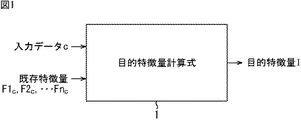••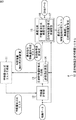•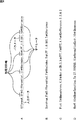•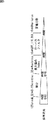•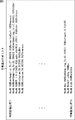•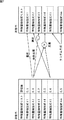•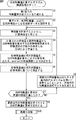•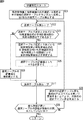•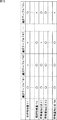•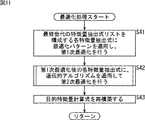•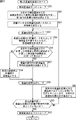•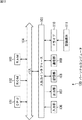## Description

The present invention relates to an information processing device, an information processing method, and a program, and in particular, an information processing device and information for automatically constructing a feature amount calculation algorithm capable of calculating a feature amount of content data such as music data. The present invention relates to a processing method and a program.

Conventionally, a feature amount calculation algorithm capable of outputting a feature amount of the input data (speed, brightness, liveliness, etc. when the input data is song data) using music data or image data as input data. An invention of automatically constructing using a genetic search method has been proposed (see, for example, Patent Document 1).

International Publication WO2007 / 049641

However, the feature quantity calculation algorithm automatically constructed by the conventional technique often includes redundant operations as compared with the feature quantity computation algorithm constructed manually, and as a result, the feature quantity calculation algorithm corresponding to the input data is obtained. In some cases, the time required to obtain the calculation becomes long.

In addition, among the developers of feature quantity calculation algorithms, it is considered that it is effective for the construction of a feature quantity calculation algorithm among the existing feature quantities that can be obtained without calculating by the feature quantity calculation algorithm to be developed. However, no method has been proposed for constructing a feature calculation algorithm.

Hereinafter, in the present specification, an existing feature quantity that can be obtained without being calculated by a feature quantity calculation algorithm to be automatically constructed is referred to as an existing feature quantity. On the other hand, a feature quantity to be obtained by a feature quantity calculation algorithm is referred to as a target feature quantity.

The present invention has been made in view of such circumstances, and automatically constructs a feature amount calculation algorithm capable of calculating a target feature amount corresponding to input data using an existing feature amount corresponding to input data. It is what you want to do.

An information processing apparatus according to one aspect of the present invention includes a genetic algorithm for generating a next generation gene by performing selection processing, crossover processing, mutation processing, and random generation processing on a previous generation gene. A feature comprising a plurality of operators in an information processing apparatus that uses input data and an existing feature amount corresponding to the input data as input and generates a target feature amount calculation formula that outputs the target feature amount corresponding to the input data The next generation feature quantity extraction formula list including a plurality of quantity extraction formulas is regarded as the gene as the plurality of feature quantity extraction formulas included in the previous generation feature quantity extraction formula list, and is calculated by a predetermined evaluation value calculation method. A feature quantity extraction formula list generated by updating the feature quantity extraction formula list of the previous generation using the genetic algorithm based on the evaluation value of the feature quantity extraction formula A generation unit, to each feature quantity extraction formulas included in the feature amount extraction expression list of the generated current generation, by entering the actual data supplied as teacher data, a plurality of feature amounts corresponding to the real data The feature quantity calculating means for calculating and the plurality of feature quantities corresponding to the calculated actual data are used, and the existing feature quantities corresponding to the actual data supplied as teacher data are also equivalent to the plurality of feature quantities And a target feature quantity calculation formula generating means for generating the target feature quantity calculation formula by predetermined machine learning for estimating the target feature quantity corresponding to the actual data supplied as teacher data, and the feature quantity the evaluation value of each feature quantity extraction formulas included in the extraction formula list, the predetermined evaluation value meter for calculating a generated value that indicates the goodness of true to the teacher data of the target feature quantity calculation equation Including an evaluation value calculating means for calculating by the method.
The target feature quantity calculation formula generation means is configured by linearly combining a plurality of feature quantities f calculated by substituting actual data into each feature quantity extraction formula, an existing feature quantity F included in teacher data, and a coefficient b. When the target feature quantity calculation formula is expressed, the target feature quantity calculation formula is generated by performing linear discrimination or linear regression using the teacher data, and the evaluation value calculation means includes the feature quantity extraction formula As the evaluation value, a total contribution rate obtained by summing up the contribution rates of the feature quantity extraction formulas with respect to each target feature quantity extraction formula to be generated may be calculated, or an average value of pearson correlation coefficients may be calculated. it can.

The target feature quantity calculation formula generation means includes: calculating a part of the plurality of feature quantities corresponding to the calculated actual data and a plurality of existing feature quantities corresponding to the actual data supplied as teacher data. optionally some, and, equally used, a predetermined machine learning for estimating the target feature amount corresponding to the actual data supplied as teacher data, to generate the target feature quantity calculation equation Can be.

The evaluation value calculation means is configured to calculate each feature quantity extraction formula included in the feature quantity extraction formula list based on a contribution rate in the target feature quantity calculation formula of the plurality of feature quantities corresponding to the calculated actual data. The evaluation value can be calculated.

An information processing method according to one aspect of the present invention includes a genetic algorithm for generating a next generation gene by performing selection processing, crossover processing, mutation processing, and random generation processing on a previous generation gene. In an information processing method of an information processing apparatus for generating a target feature quantity calculation formula that uses input data and an existing feature quantity corresponding to the input data as input and outputs a target feature quantity corresponding to the input data, a plurality of operations A feature quantity extraction formula list including a plurality of feature quantity extraction formulas consisting of children is randomly generated, and each feature quantity extraction formula included in the generated feature quantity extraction formula list of the current generation is supplied as teaching data. Data is input, a plurality of feature amounts corresponding to the actual data are calculated, the plurality of feature amounts corresponding to the calculated actual data are used, and teacher data As a result of predetermined machine learning for estimating the target feature amount corresponding to the actual data supplied as teacher data, using the existing feature amount corresponding to the actual data supplied as the same as the plurality of feature amounts. , A value indicating the goodness of applying the evaluation value of each feature quantity extraction formula included in the feature quantity extraction formula list to the teacher data of the generated target feature quantity calculation formula, generating the target feature quantity calculation formula The feature value extraction formula list of the next generation is regarded as a plurality of feature amount extraction formulas included in the feature quantity extraction formula list of the previous generation as the gene, generating by updating the characteristic amount extraction expression list of the previous generation using the genetic algorithm based on the calculated evaluation value of the feature quantity extraction formula by the evaluation value calculation method Including the step.

The program according to one aspect of the present invention uses a genetic algorithm that generates a next generation gene by performing selection processing, crossover processing, mutation processing, and random generation processing on the previous generation gene, A program for controlling an information processing apparatus for generating a target feature quantity calculation formula that outputs an input feature and an existing feature quantity corresponding to the input data and outputs a target feature quantity corresponding to the input data. A feature quantity extraction formula list including a plurality of feature quantity extraction formulas consisting of operators is randomly generated, and is supplied as teacher data to each feature quantity extraction formula included in the generated feature quantity extraction formula list of the current generation Input actual data, calculate a plurality of feature amounts corresponding to the actual data, use the plurality of feature amounts corresponding to the calculated actual data, and A predetermined machine for estimating a target feature amount corresponding to the actual data supplied as teacher data by using an existing feature amount corresponding to the actual data supplied as data as well as the plurality of feature amounts By learning, the target feature quantity calculation formula is generated, and the evaluation value of each feature quantity extraction formula included in the feature quantity extraction formula list is applied to the teacher data of the generated target feature quantity calculation formula. Calculated by a predetermined evaluation value calculation method to be calculated as a value to be shown , the next generation feature quantity extraction formula list is regarded as a plurality of feature quantity extraction formulas included in the previous generation feature quantity extraction formula list as the gene, generated by updating the characteristic amount extraction expression list of the previous generation using the genetic algorithm based on the evaluation value of the predetermined evaluation value calculation method the feature quantity extraction formula calculated by To execute a process including that step by a computer in an information processing apparatus.

In one aspect of the present invention, a feature quantity extraction formula list including a plurality of feature quantity extraction formulas including a plurality of operators is randomly generated, and each feature quantity included in the generated current generation feature quantity extraction formula list Actual data supplied as teacher data is input to the extraction formula, and a plurality of feature amounts corresponding to the actual data are calculated. In addition, a plurality of feature amounts corresponding to the calculated actual data are used, and an existing feature amount corresponding to the actual data supplied as the teacher data is also used in the same manner as the plurality of feature amounts and supplied as the teacher data. A target feature amount calculation formula is generated by predetermined machine learning for estimating the target feature amount corresponding to the actual data. Further, evaluation values of the feature quantity extraction formulas included in the feature quantity extraction formula list, the predetermined evaluation value calculating method for calculating a generated value that indicates the goodness of true to the teacher data of the target feature quantity calculation equation The feature value extraction formula calculated by the predetermined evaluation value calculation method is calculated and the feature value extraction formula list of the next generation regards the plurality of feature value extraction formulas included in the feature quantity extraction formula list of the previous generation as genes. feature amount extraction expression list of the previous generation with a genetic algorithm based on the evaluation value of is generated by being updated.

According to one aspect of the present invention, it is possible to automatically construct a feature amount calculation algorithm that can calculate a target feature amount corresponding to input data using an existing feature amount of the input data.

Hereinafter, specific embodiments to which the present invention is applied will be described in detail with reference to the drawings.

The target feature quantity formula construction system 10 (FIG. 3) to which the present invention is applied includes input data C and a plurality of existing feature quantities F1 C , F2 C ,..., Fn C as shown in FIG. The target feature quantity calculation formula 1 for outputting a plurality of target feature quantities I corresponding to the input data is generated by machine learning using a plurality of supplied teacher data.

FIG. 2 shows the data structure of teacher data. That is, the teacher data T i (i = 1,2, ··· , L) is the actual data D i which is the same type of data as the input data C, a plurality of existing feature amounts F1 i corresponding to the actual data D i To Fn i and a plurality of target feature amounts I1 i to Ik i corresponding to the actual data D i .

Existing feature amount F1 i to Fn i is a value indicating the characteristics of the actual data D i detected by existing methods from the actual data D i. Target feature amount I1 i through Ik i is a value indicating the characteristics of the actual data D i undetectable by conventional methods, for example, the actual data D i is monitored to a plurality of human digitizing impressions obtained The value determined by

As shown in FIG. 2, when there are k types of target feature amounts, the target feature amount calculation formula construction system 10 generates k target feature amount calculation formulas.

Note that the input data C may be multidimensional data, and the type thereof is arbitrary. For example, music data having a time dimension and a channel dimension, image data having an X dimension, a Y dimension, and a pixel dimension, moving image data obtained by adding a time dimension to image data, and the like can be used as the input data C. .

In the following description, an example in which music data is input data C will be described. Examples of a plurality of existing feature amounts corresponding to music data include tempo, speed, tempo fluctuation, and the like. Also, examples of the target feature amount corresponding to the music data include the brightness and speed of the music data, the number of musical instruments, and the like.

FIG. 3 shows a configuration example of the target feature quantity calculation formula construction system 10 to which the present invention is applied. The target feature quantity calculation formula construction system 10 generates a feature quantity extraction formula list consisting of a plurality of feature quantity extraction formulas, updates a feature quantity extraction formula list generation unit 11, and adds teacher data Ti to each generated feature quantity extraction formula. The feature amount calculation unit 12 that calculates the feature amount by substituting the actual data Di, the feature amount corresponding to the teacher data T i calculated by the feature amount calculation unit 12, and the existing feature amounts F1 i to Fn of the teacher data T i target feature amount calculation formula generation unit 13 for calculating the evaluation value of each feature extraction equation as to generate by machine learning target feature amount calculation formula i from the target feature amount I1 i through Ik i teacher data T i can be estimated, respectively, The final generation feature quantity extraction formula list and the target feature quantity calculation formula that are finally updated are configured by an optimization unit 15 that optimizes the formula.

The feature quantity extraction formula list generation unit 11 randomly generates a plurality of feature quantity extraction formulas constituting the first generation feature quantity extraction formula list and outputs them to the feature quantity calculation unit 12.

Here, the feature quantity extraction formula generated by the feature quantity extraction formula list generation unit 11 will be described with reference to FIG. 4A to 4D show examples of feature quantity extraction formulas, respectively.

In the feature quantity extraction formula, the type of input data is described at the left end, and the right side of the type of input data is described according to the order in which one or more types of operators are calculated. Each operator includes a process symmetry axis and parameters as appropriate.

Operator types include mean value (Mean), fast Fourier transform (FFT), standard deviation (StDev), appearance rate (Ratio), low pass filter (LPF), high pass filter (HPF), absolute value (ABS), 2 Examples include power (Sqr), square root (Sqrt), normalization (Differential), integration (Integrate), maximum value (MaxIndex), unbiased variance (UVariance), and downsampling (DownSampling). Depending on the determined operator, the process symmetry axis may be fixed. In this case, the process symmetry axis fixed to the parameter is adopted. When an operator who needs a parameter is determined, the parameter is also determined to be a random value or a preset value.

For example, in the case of the feature quantity extraction formula shown in FIG. 4A, 12TonesM is input data, and 32 # Differential, 32 # MaxIndex, 16 # LPF_1; O.861, 16 # UVariance are operators. In addition, 32 #, 16 #, etc. in each operator indicate processing symmetry axes.

Here, 12TonesM indicates that the pitch analysis of monaural PCM (pulse coded modulation sound source) waveform data is performed along the time axis, 48 # is the channel axis, 32 # is the frequency axis and the pitch axis, 16 # indicates a time axis. 0.861 in the operator is a parameter in the low-pass filter process, and indicates, for example, a threshold value of the frequency to be transmitted.

The type of input data of each feature quantity extraction formula constituting the first generation feature quantity extraction formula list is the same as that of the input data C, and the number and types of operators are randomly determined. As shown in FIG. 5, as the calculation corresponding to a plurality of operators is sequentially executed, the number of retained dimensions in the calculation result decreases sequentially, and the final calculation result of the feature quantity extraction formula is obtained. Is a scalar, or the number of dimensions thereof is set to a predetermined small value (for example, 1, 2, etc.).

As is clear from the examples shown in FIGS. 4A to 4D, the feature amount calculated by the feature amount extraction formula is an existing concept such as a tempo for music data and a pixel histogram for image data. It is not a value that is judged to be meaningful. That is, the feature quantity calculated by the feature quantity extraction formula may be merely a calculation result when the input data is substituted into the feature quantity extraction formula.

Hereinafter, it is assumed that the feature quantity extraction formula list generated by the feature quantity extraction formula list generation unit 11 includes m feature quantity extraction formulas f1 to fm as shown in FIG. WavM, which is input data of the feature quantity extraction formulas f1 to fm, is monaural PCM waveform data, and possession dimensions are a time axis and a channel axis.

Returning to FIG. The feature quantity extraction formula list generation unit 11 also generates a feature quantity extraction formula list for the second and subsequent generations by updating the feature quantity extraction formula list of the previous generation according to a genetic algorithm (GA).

Here, the genetic algorithm refers to an algorithm for generating the next generation gene from the current generation gene by selection processing, crossover processing, mutation processing, and random generation processing. Specifically, each of the plurality of feature quantity extraction formulas constituting the feature quantity extraction formula list is regarded as a gene, and selection processing is performed according to the evaluation values of the plurality of feature quantity extraction formulas constituting the current generation feature quantity extraction formula list Then, a cross process, a mutation process, and a random generation process are performed to generate a next generation feature quantity extraction formula list.

That is, for example, as shown in FIG. 7, in the selection process, a feature quantity extraction formula f2 having a high evaluation value is selected from a plurality of feature quantity extraction formulas constituting the current generation feature quantity extraction formula list to generate the next generation. Include in feature quantity extraction formula list. In the intersection processing, among the plurality of feature quantity extraction formulas constituting the current generation feature quantity extraction formula list, a plurality of feature quantity extraction formulas f2 and f5 having a high evaluation value are crossed (combined) to obtain a feature quantity extraction formula. Generate and include in the next generation feature quantity extraction formula list.

In the mutation processing, feature quantity extraction is performed by partially mutating (changing) a feature quantity extraction formula f2 having a high evaluation value among a plurality of feature quantity extraction formulas constituting the current generation feature quantity extraction formula list. Generate the formula and include it in the next generation feature extraction formula list. In the random generation process, a new feature quantity extraction formula is randomly generated and included in the next-generation feature quantity extraction formula list.

Returning to FIG. Feature amount calculation unit 12, to each feature quantity extraction formula f1 to fm constituting the supplied feature quantity extraction formula list from the feature extraction equation list generator 11, the actual data D i teacher data T i fed Substituting and calculating the feature quantity for the teacher data T i , measuring the calculation time required to calculate each of the feature quantity extraction formulas f1 to fm, and substituting L actual data D i different for each feature quantity extraction formula To calculate the average calculation time. The calculated feature value and the calculated average calculation time are supplied to the target feature value calculation formula generation unit 13.

As described above, since the number of training data T i L, the number of feature quantity extraction formulas constituting the feature quantity extraction formula list is m, the feature quantity calculator 12, (L × m) pieces of feature quantity Will be calculated. Hereinafter, calculation is performed by substituting the actual data D i of the teacher data T i (i = 1, 2,..., L) into the feature quantity extraction formula fj (j = 1, 2,..., M). The feature amount is described as fj [T i ].

Every time (L × m) feature quantities fj [T i ] corresponding to the current generation feature quantity extraction formula list are supplied from the feature quantity calculation section 12, the target feature quantity calculation formula generation unit 13, for example, The target feature value I1 C corresponding to the input data C is obtained by linear combination of the existing feature values F1 C to Fn C corresponding to the input data C and the feature values f1 [C] to fm [C] shown in (1). The target feature amount calculation formula to be estimated is (L × m) feature amounts fj [T i ], which are the calculation results of the feature amount calculation unit 12, and (L × n) existing features included in the teacher data T i. It is generated by machine learning (linear discrimination or regression with feature selection) using the quantities F1 i to Fn i and L target feature quantities I1 i included in the teacher data T i .
Target feature value I1 C = b 0 + b 1 · F 1 C + b 2 · F 2 C +... + B n · Fn C
+ B n + 1 · f1 [C] + b n + 2 · f2 [C] +... + B n + m · fm [C]
... (1)

In Equation (1), b 0 is an intercept, and b 1 , b 2 ,..., B n + m are linear coupling coefficients. In the target feature quantity calculation formula actually generated by the target feature quantity calculation formula generation unit 13, all of the existing feature quantities F1 C to Fn C and the feature quantities f1 [C] to fm [C] are used. Rather, it is used selectively. In this case, the linear combination coefficients corresponding to the existing feature amounts F1 C to Fn C and feature amounts f1 [C] through fm [C] not used are 0.

Each Similarly, existing feature amounts F1 C to Fn C and the feature amount f1 [C] corresponds to the input data C to fm [C] and the target feature amount corresponding to the input data C by a linear combination I2 C to the Ik C A target feature amount calculation formula that can be calculated is also generated.

Accordingly, the target feature quantity calculation formula generation unit 13 generates k target feature quantity calculation formulas.

Then, when the generated target feature quantity calculation formula reaches a desired accuracy, or when a predetermined instruction is given from the user, the feature quantity extraction formula list at that time is used as the feature generation extraction formula list of the last generation, It supplies to the optimization part 15 with the target feature-value calculation formula.

In addition, the target feature quantity calculation formula generation unit 13 uses the built-in evaluation value calculation unit 14 to calculate the evaluation value of each feature quantity extraction formula constituting the current generation feature quantity extraction formula list. That is, the evaluation value calculation unit 14 calculates the contribution rate of each feature quantity extraction formula in each of the k target feature quantity calculation formulas, and calculates the total contribution rate that is the sum of the calculated k contribution rates as the current generation. Are determined as evaluation values of the feature quantity extraction formulas constituting the feature quantity extraction formula list.

Here, the calculation method of a contribution rate is demonstrated using following Formula (2). Incidentally, formula (2), the existing characteristic quantity of equation (1) F1 C, ···, Fn C a feature amount f1 [C], · · ·, the fm [C], X 1, X 2, · .., replaced with X n + m
Target feature value I1 C = b 0 + b 1 · X 1 + b 2 · X 2 +... + B n + m · X n + m
... (2)

The contribution ratio (X M ) of X M (M = 1, 2,..., N + m) in the calculation of the target feature quantity I1 C according to the expression (2) is calculated by the following expression (3).

(X M ) = b M × Stdev (X M ) / StDev (I1) × Correl (X M , I1) (3)

Here, StDev (X M ) indicates a standard deviation of L X M used for machine learning.

Stdev (I1) indicates a standard deviation of L target feature values I1 i included in the teacher data T i used for machine learning.

Correl (X M, I1) shows the correlation coefficient of pearson of using the machine learning, L-number of X M and the supervisor data T i L pieces target feature amount I1 i of contained.

Note that pearson's correlation coefficient Correl (X M , I 1) is obtained by calculating the covariance between L X M and L target feature values I 1 i by L pieces as shown in the following equation (4). It is calculated by dividing by the product of the standard deviation of X M and the standard deviation of L target feature values I1 i .
Correl (X M , I1)
= (Covariance between X M and I1 i ) / (standard deviation of X M × standard deviation of I1 i )
... (4)

Note that the evaluation value calculation unit 14 uses the evaluation values of the feature quantity extraction formulas f1 to fm constituting the current generation feature quantity extraction formula list as the feature value extraction formulas f1 in the target feature quantity calculation formula as described above. Instead of determining based on the contribution ratios of the feature quantities f1 [] to fm [] that are output values of fm to fm, the evaluation value may be determined based on the pearson correlation coefficient.

Eg, L pieces of the teacher data T L pieces of feature quantities of the actual data D i respectively calculated by substituting the feature amount extraction expression f1 of i f1 and [D i], the k kinds of L tutor data T i the pearson correlation coefficient between the target feature amount I1 i through Ik i were calculated, the calculated average value of the k pearson correlation coefficient may be set as the evaluation value feature quantity extraction formulas f1.

In addition, the evaluation value calculator 14 may calculate not only the evaluation values of the feature quantity extraction formulas f1 to fm but also the evaluation values of the existing feature quantities F1 to Fm.

Furthermore, the evaluation value calculation unit 14 is supplied with the evaluation values of the feature quantity extraction formulas f1 to fm constituting the feature quantity extraction formula list of the current generation determined as described above from the feature quantity calculation unit 12. Correction is made based on the average calculation time of each feature quantity extraction formula f1 to fm. Specifically, the evaluation value of the feature quantity extraction formula whose average calculation time is equal to or greater than a predetermined threshold is corrected to the lowest value in the setting range. Then, the corrected evaluation value is notified to the feature quantity extraction formula list generation unit 11.

By correcting the evaluation value in this way, it is possible to prevent the feature quantity extraction formula whose average calculation time is equal to or greater than a predetermined threshold from being inherited in the next-generation feature quantity extraction formula list. Therefore, it is possible to reduce the calculation load of the feature amount calculation unit 12 for the next generation and later. Note that the predetermined threshold value to be compared with the average calculation time may be automatically set according to the calculation capability of the feature amount calculation unit 12, or may be arbitrarily set by the user.

The optimization unit 15 includes a feature quantity extraction formula optimization unit 16 that optimizes the feature quantity extraction formulas f1 to fm constituting the final generation feature quantity extraction formula list supplied from the target feature quantity calculation formula generation unit 13; A target feature quantity calculation formula restructuring unit 17 for reconstructing the target feature quantity calculation formula using the optimized feature quantity extraction formulas f1 to fm is incorporated.

The feature quantity extraction formula optimization unit 16 uses a combination of redundant operations registered in advance (hereinafter referred to as an optimization pattern) from the feature quantity extraction formulas f1 to fm constituting the final generation feature quantity extraction formula list. Is detected, and the first optimization is performed by replacing it with an operation having a smaller processing load than an equivalent operation result. An example of the first optimization is given below.

The optimization pattern in which two or more operators Abs for calculating an absolute value are continuous is optimized by replacing it with one operator Abs since the second and subsequent operators Abs are redundant.

The optimization pattern in which two or more operators Normalize indicating normalization operations are continuous is optimized by replacing the operator Normalize for the second and subsequent times with one operator Normalize.

The optimization pattern in which the operator Sqr indicating the square calculation and the operator Sqrt for calculating the square root are continued is optimized by replacing the operator Abs with a smaller calculation load than obtaining the same result.

Since an optimization pattern in which an operator Defferential indicating differential operation and an operator Integrate indicating integral operation are continuous is a useless operation, optimization is performed by deleting the operators Differential and Integrate.

Note that the optimization pattern and the optimization method are not limited to the above-described example.

Further, the feature quantity extraction formula optimizing unit 16 performs secondary optimization using the genetic algorithm so that equivalent calculation results can be obtained for each feature quantity extraction formula f1 to fm in a shorter calculation time.

The target feature quantity calculation formula reconstruction unit 17 reconstructs the target feature quantity calculation formula by machine learning using the optimized feature quantity extraction formulas f1 to fm and teacher data.

Next, the operation of the target feature quantity calculation formula construction system 10 will be described with reference to the flowchart of FIG.

In step S <b> 1, the feature quantity extraction formula list generation unit 11 randomly generates m feature quantity extraction formulas constituting the first generation feature quantity extraction formula list, and includes feature quantities including the m feature quantity extraction formulas. The extraction formula list is supplied to the feature amount calculation unit 12.

In step S2, the target feature quantity calculation formula construction system 10 acquires teacher data T i (i = 1, 2,..., L). The acquired teacher data Ti is supplied to the feature quantity calculation unit 12, the target feature quantity calculation formula generation unit 13, and the optimization unit 15.

In step S <b> 3, the feature quantity calculation unit 12 includes the actual data included in the teacher data T i in each of the feature quantity extraction formulas f1 to fm constituting the feature quantity extraction formula list supplied from the feature quantity extraction formula list generation unit 11. By substituting D i , (L × m) feature quantities fj [T i ] are calculated, and the calculation time required for calculating each of the feature quantity extraction formulas f1 to fm is also measured. An average calculation time is calculated when different L pieces of actual data D i are substituted for each calculation. The calculated (L × m) feature values fj [T i ] and the average calculation time corresponding to each of the feature value extraction formulas f1 to fm are supplied to the target feature value calculation formula generation unit 13.

In step S4, the target feature quantity calculation formula generation unit 13 includes (L × m) feature quantities fj [T i ], which are the calculation results of the feature quantity calculation unit 12, and the teacher data T i (L × and a n) pieces of the existing feature amounts F1 i to Fn i, learns the feature selection with linear discriminant or regression of the desired characteristic quantity calculation equation for estimating the L-number target feature amount I1 i that are included in the training data T i.

The process of step S4 (hereinafter referred to as learning process) by the target feature quantity calculation formula generation unit 13 will be described in detail with reference to the flowchart of FIG.

In step S21, the target feature quantity calculation formula generation unit 13 generates the target feature quantity calculation formula, and the feature quantity f1 that is an output of the n existing feature quantities F1 to Fn and the m feature extraction formulas f1 to fm. Among the [] to fm [], a plurality of selection tables TB indicating what is used (selected) and not used (not selected) are randomly generated to generate a first generation selection table group. In this selection table group, a plurality of selection tables TB configured are regarded as genes, and are updated based on a genetic algorithm in the following step S29.

FIG. 10 shows an example of a selection table group including a plurality of selection tables TB to be generated. In FIG. 10, circles ○ indicate selection, and crosses X indicate non-selection.

In step S22, the target feature quantity calculation formula generation unit 13 starts the selection table group loop by paying attention to each selection table TB constituting the selection table group of the current generation one by one. In the selection table group loop, only the number of selection tables TB constituting the selection table group (x in the example of FIG. 10) is repeated.

In step S23, the target feature quantity calculation formula generation unit 13 uses the average calculation time corresponding to each of the feature quantity extraction formulas f1 to fm supplied from the feature quantity calculation unit 12, and selects the target feature quantity calculation formula using the selected selection table TB. It is determined whether or not the total of the average calculation times of the feature extraction formula fj corresponding to the feature amount fj [] being set is equal to or less than a predetermined threshold. The predetermined threshold value to be compared with the total of the average calculation time may be automatically set according to the calculation capability of the target feature quantity calculation formula generation unit 13 or the like, or may be arbitrarily set by the user. Good.

If it is determined that the total average calculation time is equal to or less than the predetermined threshold, the process proceeds to step S24.

In step S24, the target feature quantity calculation formula generation unit 13 includes (L × m) feature quantities fj [T i ], which are calculation results by the feature quantity calculation unit 12, and the teacher data T i (L × n) Among the existing feature quantities F1 i to Fn i , the target feature quantity of the number (k) of target feature quantity types is obtained by linear discrimination or regression using the one selected by the selected selection table TB. Learn the formula.

In step S25, the target feature quantity calculation formula generation unit 13 calculates the information amount reference AIC of the learning result by the process of step S24 as the evaluation value of the selected selection table TB.

If it is determined in step S23 that the total of the average calculation times of the feature extraction formula fj corresponding to the feature quantity fj [] selected by the selected selection table TB is not less than or equal to a predetermined threshold value, Advances to step S26. In step S26, the target feature quantity calculation formula generation unit 13 sets the evaluation value of the selected selection table TB as the lowest value of the setting range. In this way, by preventing the selection table whose total average calculation time is longer than the predetermined threshold from being inherited by the next generation, it is possible to prevent the time required for calculating the generated target feature amount calculation formula from being increased. Can do.

After the evaluation value of the selection table TB focused on in step S25 or step S26 is determined, the process proceeds to step S27. In step S27, the target feature quantity calculation formula generation unit 13 determines whether or not all the selection tables TB constituting the selection table group of the current generation have been noticed. The process returns to step S22, and the processes of steps S22 to S27 are repeated. If it is determined in step S27 that all the selection tables TB constituting the current generation selection table group have been noticed, the process proceeds to step S28.

In step S28, the target feature quantity calculation formula generation unit 13 determines whether or not the evaluation value of the selection table TB having the highest evaluation has been improved for a predetermined number of generations. Then, when it is determined that the evaluation value of the selection table TB having the highest evaluation has improved or the predetermined number of generations has not elapsed since it has not improved, the process proceeds to step S29.

In step S29, the target feature quantity calculation formula generation unit 13 generates the next generation selection table group by updating the current generation selection table group with a genetic algorithm based on the evaluation value of each selection table TB. The process returns to step S22, and the subsequent processes are repeated.

If it is determined in step S28 that the evaluation value of the selection table TB having the highest evaluation has not improved for a predetermined number of generations, the process proceeds to step S5 in FIG.

Through the learning process described above, the target feature quantity calculation formulas for calculating the k types of target feature quantities corresponding to the current generation feature quantity extraction formula list are generated.

In the above description, the learning process is described as using the genetic search method and AIC, but the learning process may be performed by a different method. Further, instead of using a genetic algorithm for determining whether to select or deselect an output value of an existing feature quantity or feature extraction formula, a neighborhood search may be used.

For example, in the case of using neighborhood search, learning is started as non-selection of all n feature amounts F1 to Fn and feature amounts f1 [] to fm [] that are outputs of m feature extraction formulas f1 to fm. . Then, one of the n existing feature amounts F1 to Fn and the m feature extraction formulas f1 to fm is selected as one of the feature amounts f1 [] to fm [] and the other is not selected. A selection table is generated, and each selection table is evaluated by AIC or the like. Then, a selection table having the highest evaluation, that is, a small AIC value is determined. Further, based on the selected selection table, select one of the n existing feature amounts F1 to Fn and the feature amounts f1 [] to fm [], which are outputs of the m feature extraction formulas f1 to fm. In addition, n + m selection tables are generated with the others not selected, and each selection table is evaluated by AIC or the like. The above processing may be repeated until the evaluation of AIC or the like is not improved.

Returning to FIG. In step S5, the evaluation value calculation unit 14 of the target feature quantity calculation formula generation unit 13 is a feature that is the calculation result of each of the feature quantity extraction formulas f1 to fm in the k target feature quantity calculation formulas currently generated. The contribution ratios of the quantities f1 [] to fm [] are calculated, and the total contribution ratios obtained by summing the calculated contribution ratios of k are used as the feature quantity extraction formulas f1 to fm constituting the feature quantity extraction formula list of the current generation. The evaluation value is determined.

In step S5, as described above, the evaluation values of the feature quantity extraction formulas f1 to fm constituting the current generation feature quantity extraction formula list are set to the feature quantity extraction formulas f1 to fm in the target feature quantity calculation formula, as described above. Instead of determining based on the contribution rates of the feature quantities f1 [] to fm [], which are output values, the evaluation value may be determined based on the pearson correlation coefficient.

In step S6, the evaluation value calculation unit 14 supplies the evaluation values of the feature quantity extraction formulas f1 to fm constituting the current generation feature quantity extraction formula list determined in the process of step S5 from the feature quantity calculation unit 12. The feature amount extraction formulas f1 to fm are corrected based on the average calculation time. Specifically, the evaluation value of the feature quantity extraction formula whose average calculation time is equal to or greater than a predetermined threshold is corrected to the lowest value in the setting range. Then, the corrected evaluation value is notified to the feature quantity extraction formula list generation unit 11.

In step S <b> 7, the target feature quantity calculation formula generation unit 13 determines whether or not the calculation result based on the target feature quantity calculation formula generated at the present time has reached a desired accuracy, or whether or not an end operation has been performed by the user. Determine whether. If it is determined that the calculation result has not reached the desired accuracy and the user has not performed the end operation, the process proceeds to step S8.

In step S8, the feature quantity extraction formula list generation unit 11 also generates the next generation feature quantity extraction formula list by updating the current generation feature quantity extraction formula list according to the genetic algorithm. Thereafter, the process returns to step S3, and the processes after step S3 are repeated.

If it is determined in step S7 that the calculation result based on the target feature amount calculation formula generated at the present time has reached a desired accuracy or the user has performed an end operation, the process proceeds to step S9.

In step S <b> 9, the target feature quantity calculation formula generation unit 13 converts the current generation feature quantity extraction formula list and the target feature quantity calculation formula generated at the present time into the last generation feature quantity extraction formula list and the corresponding k. This is output to the optimization unit 15 as a target feature quantity calculation formula.

In step S10, the optimization unit 15 optimizes the feature quantity extraction formulas f1 to fm in the final generation feature quantity extraction formula list input from the target feature quantity calculation formula generation unit 13, and optimizes each feature. A target feature quantity calculation formula using the quantity extraction formulas f1 to fm is reconstructed.

The process of step S10 by the optimization unit 15 will be described in detail with reference to the flowchart of FIG.

In step S41, the feature quantity extraction formula optimization unit 16 detects an optimization pattern from the feature quantity extraction formulas f1 to fm constituting the final generation feature quantity extraction formula list, and obtains an equivalent result. First-order optimization is performed by replacing the calculation with a low processing load.

In step S42, the feature quantity extraction formula optimization unit 16 uses a genetic algorithm so that each feature quantity extraction formula f1 to fm after the first optimization can be obtained in a shorter calculation time. Second-order optimization is performed.

The process of step S42 by the feature quantity extraction formula optimization unit 16 will be described in detail with reference to the flowchart of FIG.

In step S51, the feature quantity extraction formula optimization unit 16 extracts feature quantities by paying attention to the feature quantity extraction formulas f1 to fm constituting the final generation feature quantity extraction formula list after the first optimization one by one. Start an expression list loop. The feature quantity extraction formula list loop is repeated by the number m) of the feature quantity extraction formulas f1 to fm constituting the feature quantity extraction formula list.

In step 52, the feature quantity extraction formula optimizing unit 16 makes R optimization candidate formulas fj ′ r (r = 1, 2,...) Obtained by mutating a part of the feature quantity extraction formula fj of interest. R) is generated and set as the first generation optimization candidate formula group.

In step S53, the feature quantity extraction formula optimization unit 16 substitutes S pieces of evaluation data (the same type of data as the input data C) for the feature quantity extraction formula fj of interest, and S feature quantities fj []. Calculate

In step S54, the feature quantity extraction formula optimization unit 16 starts the optimization candidate formula group loop by paying attention to the R optimization candidate formulas constituting the optimization candidate formula group of the current generation one by one. The optimization candidate formula group loop is repeated as many times as the number of optimization candidate formulas constituting the optimization candidate formula group.

In step S55, the feature quantity extraction formula optimization unit 16 substitutes the S evaluation data used also in step 53 into the optimization candidate formula fj ′ r of interest, and the S feature quantities fj ′ r []. Is calculated, the calculation time when each evaluation data is substituted is measured, and the average calculation time is further calculated.

In step S56, the feature quantity extraction formula optimization unit 16 calculates the S feature quantity fj [] that is the process result of step S53 and the S feature quantity fj ′ r [] that is the process result of step S55. A pearson correlation coefficient indicating the degree of correlation is calculated, and it is determined whether or not the degree of correlation between the S feature quantities fj [] and the S feature quantities fj ′ r [] is approximately 1.0. . If it is determined that the correlation between the S feature values fj [] and the S feature values fj ′ r [] is approximately 1.0, the process proceeds to step S57.

In step S57, the feature amount extraction expression optimizing unit 16 as an evaluation value of the optimizing candidate expression fj 'r of interest, to set the average inverse of the computation time calculated in the processing in step S55.

In step S56, it is determined whether or not the degree of correlation between the S feature values fj [] and the S feature values fj ′ r [] is not approximately 1.0. If it is determined that the degree of correlation between the S feature values fj [] and the S feature values fj ′ r [] is not approximately 1.0, the process proceeds to step S58.

In step S58, the feature amount extraction expression optimizing unit 16 sets the minimum value of the range to the evaluation value of the optimizing candidate expression fj 'r of interest.

In processing or the processing in step S58 in step S57, the after evaluation value of the optimizing candidate expression fj 'r of interest has been determined, the process proceeds to step S59. In step S59, the feature amount extraction expression optimizing unit 16 determines whether attention all optimizing candidate expression fj 'r constituting the optimizing candidate expression group of the current generation, optimization candidates that are not focused If the expression fj ′ r remains, the process returns to step S54, and the processes of steps S54 to S59 are repeated. Then, in step S59, the case where it is determined that the attention of all optimizing candidate expression fj 'r constituting the optimizing candidate expression group of the current generation, the processing proceeds to step S60.

In step S60, the feature quantity extraction formula optimization unit 16 determines whether or not the evaluation value of the optimization candidate formula having the best evaluation has improved for a predetermined number of generations. If it is determined that the evaluation value of the optimization candidate formula having the best evaluation has improved, or it has been determined that the predetermined number of generations has not elapsed since it has not improved, the process proceeds to step S61.

In step S61, the feature amount extraction expression optimizing unit 16, the optimizing candidate expression group of the current generation based on the evaluation value of each optimizing candidate expression fj 'r, by updating the genetic algorithm, the next generation of optimal Generate a group of candidate expressions. However, the next generation optimization candidate formula group includes the optimization candidate formula with the highest evaluation of the previous generation, and the remaining (R-1) optimization candidate formulas have the highest evaluation of the previous generation. A part of the good optimization candidate formula and the feature quantity extraction formula fj to be noticed are mutated and generated. The processing returns to step S54, and the subsequent processing is repeated.

If it is determined in step S60 that the evaluation value of the optimization candidate formula having the best evaluation has not improved for a predetermined number of generations, the process proceeds to step S62.

As described above, the second optimization has been performed on one of all the feature quantity extraction formulas constituting the feature quantity extraction formula list of the final generation after the first optimization by the processing of steps S52 to S60. become.

In step S62, the feature quantity extraction formula optimization unit 16 determines whether or not all feature quantity extraction formulas f1 to fm constituting the final generation feature quantity extraction formula list after the first optimization have been noticed, When the feature quantity extraction formula fj that is not focused remains, the process returns to step S51, and the processes of steps S51 to S62 are repeated. If it is determined in step S62 that all feature quantity extraction formulas f1 to fm constituting the final generation feature quantity extraction formula list after the first optimization are noticed, the final result after the first optimization Since the second optimization has been performed on all the feature quantity extraction formulas f1 to fm constituting the generation feature quantity extraction formula list, the process proceeds to step S43 in FIG.

Returning to FIG. In step S43, the target feature quantity calculation formula reconstruction unit 17 generates a target feature quantity calculation formula by statistical analysis and machine learning based on the second-optimized feature quantity extraction formulas f1 to fm and the teacher data. The k target feature amount calculation formulas supplied from the unit 13 are reconstructed. Then, the final feature quantity extraction formula list and the target feature quantity calculation formula are output, and the operation of the target feature quantity calculation formula construction system 10 is terminated.

This is the end of the description of the operation of the target feature quantity calculation formula construction system 10.

As described above, according to the target feature quantity calculation formula construction system 10 to which the present invention is applied, the target feature quantity formula is automatically constructed using the existing feature quantity, so only the calculation result of the feature quantity formula is obtained. The number of feature quantity extraction formulas that tend to be redundant can be reduced as compared with the case where the target feature quantity computation formula is constructed. Therefore, it is possible to reduce the amount of calculation processing when calculating the target feature value from the target feature value calculation formula.

In addition, according to the target feature amount calculation formula construction system 10 to which the present invention is applied, since the gene of the feature amount calculation formula having a long calculation time is not left behind in the genetic algorithm, the calculation time is limited. The target feature quantity calculation formula can be constructed. In addition, the construction time of the target feature amount calculation formula can be shortened.

Furthermore, according to the target feature quantity calculation formula construction system 10 to which the present invention is applied, the feature quantity extraction formula constituting the feature quantity extraction formula list is optimized, so the target feature quantity is calculated from the target feature quantity calculation formula. The amount of computation processing can be reduced.

By the way, the above-described series of processing can be executed by hardware or can be executed by software. When a series of processing is executed by software, a program constituting the software may execute various functions by installing a computer incorporated in dedicated hardware or various programs. For example, it is installed from a program recording medium in a general-purpose personal computer or the like.

FIG. 13 is a block diagram illustrating a hardware configuration example of a computer that executes the above-described series of processing by a program.

In this computer 100, a CPU (Central Processing Unit) 101, a ROM (Read Only Memory) 102, and a RAM (Random Access Memory) 103 are connected to each other by a bus 104.

An input / output interface 105 is further connected to the bus 104. The input / output interface 105 includes an input unit 106 including a keyboard, a mouse, and a microphone, an output unit 107 including a display and a speaker, a storage unit 108 including a hard disk and nonvolatile memory, and a communication unit 109 including a network interface. A drive 110 for driving a detachable recording medium 111 such as a magnetic disk, an optical disk, a magneto-optical disk, or a semiconductor memory is connected.

In the computer configured as described above, the CPU 101 loads, for example, the program stored in the storage unit 108 to the RAM 103 via the input / output interface 105 and the bus 104 and executes the program. Is performed.

The program executed by the computer may be a program that is processed in time series in the order described in this specification, or in parallel or at a necessary timing such as when a call is made. It may be a program for processing.

The program may be processed by a single computer, or may be distributedly processed by a plurality of computers. Furthermore, the program may be transferred to a remote computer and executed.

Further, in this specification, the system represents the entire apparatus constituted by a plurality of apparatuses.

The embodiment of the present invention is not limited to the above-described embodiment, and various modifications can be made without departing from the gist of the present invention.

It is a figure explaining the objective feature-value calculation formula produced | generated by the target feature-value calculation formula construction system to which this invention was applied. It is a figure which shows the data structure of teacher data. It is a block diagram which shows the structural example of the target feature-value calculation formula construction system to which this invention was applied. It is a figure which shows the example of a feature-value extraction formula. It is a figure explaining the structure of a feature-value extraction formula. It is a figure which shows the example of a feature-value extraction formula list. It is a figure for demonstrating a genetic algorithm. It is a flowchart explaining operation | movement of the target feature-value calculation formula construction system to which this invention was applied. It is a flowchart detailing step S4 of FIG. It is a figure which shows the example of a selection table group. It is a flowchart detailing step S10 of FIG. 12 is a flowchart detailing step S42 in FIG. 11. It is a block diagram which shows the structural example of a computer.

Explanation of symbols

DESCRIPTION OF SYMBOLS 10 Objective feature quantity formula construction system, 11 Feature quantity extraction formula list generation part, 12 Feature quantity calculation part, 13 Objective feature quantity calculation formula generation part, 14 Evaluation value calculation part, 15 Optimization part, 16 Feature quantity extraction formula optimization Unit, 17 target feature value calculation formula reconstruction unit, 101 CPU, 111 recording medium

## Claims (6)

1. Performs selection processing, crossover processing, mutation processing, and random generation processing on the previous generation gene, and uses a genetic algorithm that generates the next generation gene, using the input data and the existing data corresponding to the input data. In an information processing apparatus for generating a target feature amount calculation formula that outputs a target feature amount corresponding to the input data, using the feature amount as an input
A next-generation feature quantity extraction formula list including a plurality of feature quantity extraction formulas composed of a plurality of operators is regarded as a plurality of feature quantity extraction formulas included in the previous generation feature quantity extraction formula list as a predetermined evaluation. A feature quantity extraction formula list generation means for generating the feature quantity extraction formula list of the previous generation using the genetic algorithm based on the evaluation value of the feature quantity extraction formula calculated by the value calculation method;
A feature quantity for calculating a plurality of feature quantities corresponding to the actual data by inputting actual data supplied as teacher data to each feature quantity extraction formula included in the generated feature quantity extraction formula list of the current generation Calculation means;
The plurality of feature amounts corresponding to the calculated actual data are used, and the existing feature amounts corresponding to the actual data supplied as teacher data are also used as the plurality of feature amounts and supplied as teacher data. A target feature amount calculation formula generating means for generating the target feature amount calculation formula by predetermined machine learning for estimating the target feature amount corresponding to the actual data;
The predetermined evaluation value calculation method for calculating the evaluation value of each feature quantity extraction formula included in the feature quantity extraction formula list as a value indicating goodness of application of the generated target feature quantity calculation formula to teacher data An information processing apparatus comprising: evaluation value calculation means for calculating by
2. The target feature quantity calculation formula generation means performs the above-described linear feature combination of a plurality of feature quantities f calculated by substituting actual data into each feature quantity extraction formula, and the existing feature quantity F included in the teacher data and the coefficient b. When the target feature amount calculation formula is expressed, the target feature amount calculation formula is generated by performing linear discrimination or linear regression using the teacher data,
The evaluation value calculation means calculates a total contribution ratio that is a sum of contribution ratios of the feature quantity extraction formula for each generated target feature quantity extraction formula as the evaluation value of the feature quantity extraction formula, or a pearson phase The information processing apparatus according to claim 1, wherein an average value of the number of relationships is calculated.
3. The target feature quantity calculation formula generation means includes: calculating a part of the plurality of feature quantities corresponding to the calculated actual data and a plurality of existing feature quantities corresponding to the actual data supplied as teacher data. The target feature quantity calculation formula is generated by predetermined machine learning for estimating the target feature quantity corresponding to the actual data supplied as teacher data by using some and selectively. The information processing apparatus according to claim 2.
4. The evaluation value calculation means is configured to calculate each feature quantity extraction formula included in the feature quantity extraction formula list based on a contribution rate in the target feature quantity calculation formula of the plurality of feature quantities corresponding to the calculated actual data. The information processing apparatus according to claim 2, wherein the evaluation value is calculated.
5. Performs selection processing, crossover processing, mutation processing, and random generation processing on the previous generation gene, and uses a genetic algorithm that generates the next generation gene, using the input data and the existing data corresponding to the input data. In an information processing method of an information processing apparatus for generating a target feature amount calculation formula that outputs a target feature amount corresponding to the input data, using the feature amount as an input,
A feature quantity extraction formula list including a plurality of feature quantity extraction formulas composed of a plurality of operators is randomly generated.
Each feature quantity extraction formulas included in the generated current generation of the feature quantity extraction formula list, and enter the actual data supplied as teacher data, calculates a plurality of feature amounts corresponding to the real data,
The plurality of feature amounts corresponding to the calculated actual data are used, and the existing feature amounts corresponding to the actual data supplied as teacher data are also used as the plurality of feature amounts and supplied as teacher data. The target feature quantity calculation formula is generated by predetermined machine learning for estimating the target feature quantity corresponding to the actual data,
Calculated by a predetermined evaluation value calculation method for calculating an evaluation value of each feature quantity extraction formula included in the feature quantity extraction formula list as a value indicating goodness of the generated target feature quantity calculation formula to teacher data And
The feature quantity extraction formula calculated by the predetermined evaluation value calculation method, considering the feature quantity extraction formula list of the next generation as a plurality of feature quantity extraction formulas included in the feature quantity extraction formula list of the previous generation. An information processing method including a step of generating by updating the feature quantity extraction formula list of the previous generation using the genetic algorithm based on the evaluation value of a formula.
6. Performs selection processing, crossover processing, mutation processing, and random generation processing on the previous generation gene, and uses a genetic algorithm that generates the next generation gene, using the input data and the existing data corresponding to the input data. A program for controlling an information processing apparatus that generates a target feature value calculation formula that outputs a target feature value corresponding to the input data, using a feature value as an input,
A feature quantity extraction formula list including a plurality of feature quantity extraction formulas composed of a plurality of operators is randomly generated.
Input actual data supplied as teacher data to each feature quantity extraction formula included in the generated feature quantity extraction formula list of the current generation, and calculate a plurality of feature quantities corresponding to the actual data,
The plurality of feature amounts corresponding to the calculated actual data are used, and the existing feature amounts corresponding to the actual data supplied as teacher data are also used as the plurality of feature amounts and supplied as teacher data. The target feature quantity calculation formula is generated by predetermined machine learning for estimating the target feature quantity corresponding to the actual data,
The evaluation values of the feature quantity extraction formulas included in the feature quantity extraction formula list, calculated by a predetermined evaluation value calculating method for calculating a generated value that indicates the goodness of true to the teacher data of the target feature quantity calculation equation And
The feature quantity extraction formula calculated by the predetermined evaluation value calculation method, considering the feature quantity extraction formula list of the next generation as a plurality of feature quantity extraction formulas included in the feature quantity extraction formula list of the previous generation. A program that causes a computer of an information processing device to execute processing including a step of generating by updating the feature quantity extraction formula list of a previous generation using the genetic algorithm based on the evaluation value of a formula.
JP2007273417A 2007-10-22 2007-10-22 Information processing apparatus, information processing method, and program Expired - Fee Related JP4433323B2 (en)

## Priority Applications (1)

Application Number Priority Date Filing Date Title
JP2007273417A JP4433323B2 (en) 2007-10-22 2007-10-22 Information processing apparatus, information processing method, and program

## Applications Claiming Priority (4)

Application Number Priority Date Filing Date Title
JP2007273417A JP4433323B2 (en) 2007-10-22 2007-10-22 Information processing apparatus, information processing method, and program
US12/243,690 US8131657B2 (en) 2007-10-22 2008-10-01 Information processing device, information processing method, and program
CN 200810170287 CN101419610B (en) 2007-10-22 2008-10-20 Information processing device, information processing method
US13/366,585 US8326779B2 (en) 2007-10-22 2012-02-06 Information processing device, information processing method, and program

## Publications (2)

Publication Number Publication Date
JP2009104274A JP2009104274A (en) 2009-05-14
JP4433323B2 true JP4433323B2 (en) 2010-03-17

# Family

## Family Applications (1)

Application Number Title Priority Date Filing Date
JP2007273417A Expired - Fee Related JP4433323B2 (en) 2007-10-22 2007-10-22 Information processing apparatus, information processing method, and program

## Country Status (2)

JP (1) JP4433323B2 (en)
CN (1) CN101419610B (en)

## Families Citing this family (3)

* Cited by examiner, † Cited by third party
Publication number Priority date Publication date Assignee Title
JP5581864B2 (en) * 2010-07-14 2014-09-03 ソニー株式会社 Information processing apparatus, information processing method, and program
JP5881048B2 (en) * 2012-09-18 2016-03-09 株式会社日立製作所 Information processing system and information processing method
CN106708609A (en) * 2015-11-16 2017-05-24 阿里巴巴集团控股有限公司 Characteristics generation method and system

## Also Published As

Publication number Publication date
JP2009104274A (en) 2009-05-14
CN101419610B (en) 2011-11-16
CN101419610A (en) 2009-04-29

## Similar Documents

Publication Publication Date Title
Deng et al. Machine learning paradigms for speech recognition: An overview
Fox et al. A sticky HDP-HMM with application to speaker diarization
Altonji et al. Cross section and panel data estimators for nonseparable models with endogenous regressors
Sigtia et al. An end-to-end neural network for polyphonic piano music transcription
Durrieu et al. Source/filter model for unsupervised main melody extraction from polyphonic audio signals
Farrell et al. Modelling transposition latencies: Constraints for theories of serial order memory
Hadjeres et al. Deepbach: a steerable model for bach chorales generation
Williams et al. Scaling up POMDPs for Dialog Management: The``Summary POMDP''Method
Fuhrer Inflation persistence
JP2006285899A (en) Learning device and learning method, creation device and creation method, and program
Mesaros et al. Detection and classification of acoustic scenes and events: Outcome of the DCASE 2016 challenge
Fiebrink et al. Human model evaluation in interactive supervised learning
US8706274B2 (en) Information processing apparatus, information processing method, and program
JP4665836B2 (en) Music classification device, music classification method, and music classification program
Roberts et al. A hierarchical latent vector model for learning long-term structure in music
JP5201602B2 (en) Sound source separation system, sound source separation method, and computer program for sound source separation
Qi et al. Music analysis using hidden Markov mixture models
Cemgil et al. Rhythm quantization for transcription
Gašić et al. Gaussian processes for pomdp-based dialogue manager optimization
Turnbull et al. Fast recognition of musical genres using RBF networks
Benetos et al. A shift-invariant latent variable model for automatic music transcription
Ni et al. An end-to-end machine learning system for harmonic analysis of music
JPH0782348B2 (en) Sub-word model generation method for speech recognition
CN101740013A (en) Information processing apparatus, sound analysis method, and program
Paiement et al. A probabilistic model for chord progressions

## Legal Events

Date Code Title Description
A131 Notification of reasons for refusal

Free format text: JAPANESE INTERMEDIATE CODE: A131

Effective date: 20090702

A521 Written amendment

Free format text: JAPANESE INTERMEDIATE CODE: A523

Effective date: 20090821

A131 Notification of reasons for refusal

Free format text: JAPANESE INTERMEDIATE CODE: A131

Effective date: 20090917

A521 Written amendment

Free format text: JAPANESE INTERMEDIATE CODE: A523

Effective date: 20091109

TRDD Decision of grant or rejection written
A01 Written decision to grant a patent or to grant a registration (utility model)

Free format text: JAPANESE INTERMEDIATE CODE: A01

Effective date: 20091203

A01 Written decision to grant a patent or to grant a registration (utility model)

Free format text: JAPANESE INTERMEDIATE CODE: A01

A61 First payment of annual fees (during grant procedure)

Free format text: JAPANESE INTERMEDIATE CODE: A61

Effective date: 20091216

R151 Written notification of patent or utility model registration

Ref document number: 4433323

Country of ref document: JP

Free format text: JAPANESE INTERMEDIATE CODE: R151

FPAY Renewal fee payment (event date is renewal date of database)

Free format text: PAYMENT UNTIL: 20130108

Year of fee payment: 3

R250 Receipt of annual fees

Free format text: JAPANESE INTERMEDIATE CODE: R250

R250 Receipt of annual fees

Free format text: JAPANESE INTERMEDIATE CODE: R250

R250 Receipt of annual fees

Free format text: JAPANESE INTERMEDIATE CODE: R250

R250 Receipt of annual fees

Free format text: JAPANESE INTERMEDIATE CODE: R250

R250 Receipt of annual fees

Free format text: JAPANESE INTERMEDIATE CODE: R250

R250 Receipt of annual fees

Free format text: JAPANESE INTERMEDIATE CODE: R250

LAPS Cancellation because of no payment of annual fees